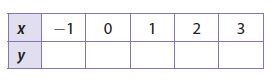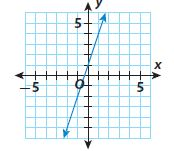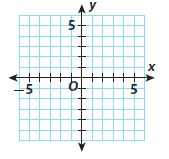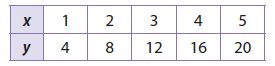Homework Explained - Math Practice 101Dear guest, you are not a registered member. As a guest, you only have read-only access to our books, tests and other practice materials.

As a registered member you can:

Registration is free and doesn't require any type of payment information. Click here to Register.
Go to page:
Chapter 4: Nonproportional Relationships; Model Quiz

4.1 Representing Linear Nonproportional Relationships

• Question 1

Complete the table using the equation $$\text{y} = 3\text{x} + 2$$.• Type below:

4.2 Determining Slope and y-intercept

• Question 2

Find the slope and y-intercept of the line in the graph.• Type below:

4.3 Graphing Linear Nonproportional Relationships

• Question 3

Graph the equation $$\text{y} = 2\text{x} - 3$$ using slope and y-intercept.• Type below:

4.4 Proportional and Nonproportional Situations

• Question 4

Does the table represent a proportional or a nonproportional linear relationship?• Question 5

Does the graph in Exercise 2 represent a proportional or a nonproportional linear relationship?

• Question 6

Does the graph in Exercise 3 represent a proportional or a nonproportional relationship?

Essential Question

• Question 7

How can you identify a linear nonproportional relationship from a table, a graph, and an equation?

• Type below:

Yes, email page to my online tutor. (if you didn't add a tutor yet, you can add one here)